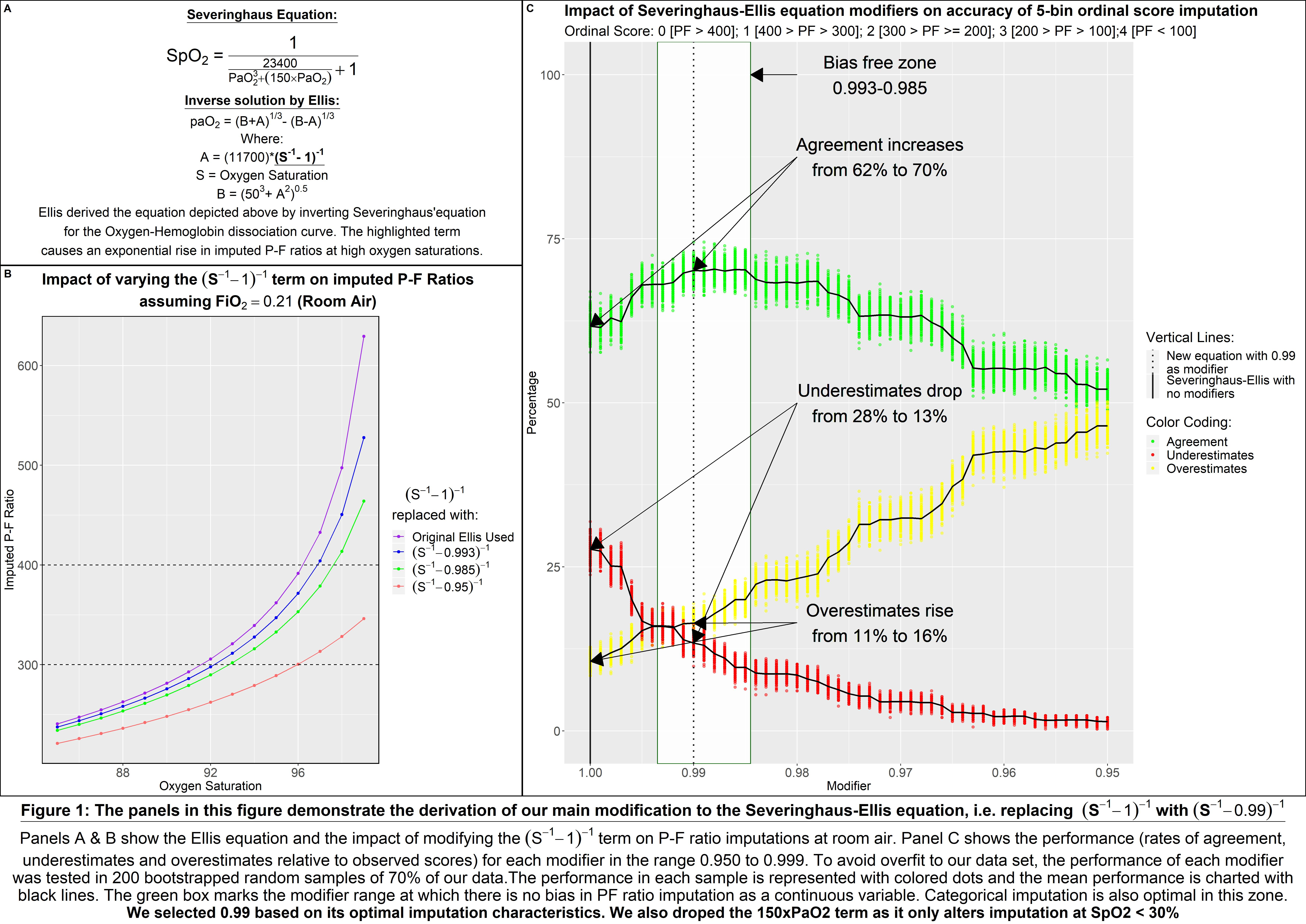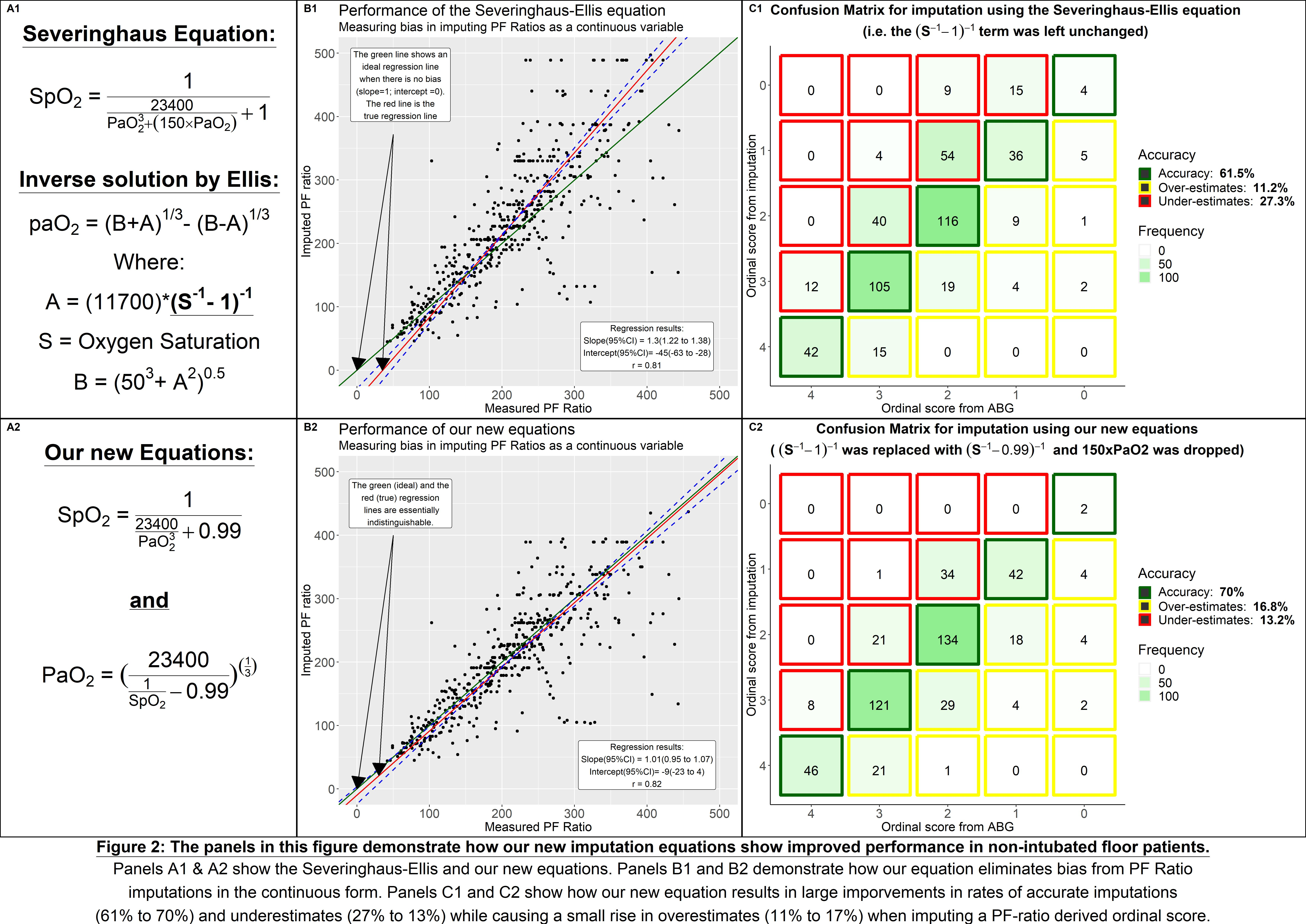Background: The ratio of partial pressure of arterial oxygen to the fraction of inspired oxygen (P:F ratio) has known clinical utility. For example, it is a key component of the sequential organ failure assesment (SOFA) score which is a validated mortality predictor and the new operational defintion of sepsis. Yet, its use is limited because measuring it requires arterial blood sampling (ABGs). An accurate estimate of the P:F ratio, assessed continuously and non-invasively, would be a valuable tool for hospitalists. Existing equations for imputing P:F ratio from oxygen saturations (SpO2) have been well-validated in ICU patients, but there is limited research assessing their accuracy in non-intubated floor patients.

Methods: We sampled non-intubated floor patients hospitalized at 1 large tertiary care center between 2013 and 2017. Patients were included if they had SpO2 charted ≤10 minutes prior to an ABG draw (N=493). As a first step, existing equations (Severinghaus-Ellis, Rice & Pandharipande) were used to impute P:F ratios from SpO2. The measured and imputed P:F ratios were compared both in their continuous form (major axis regression) and after transformation into derivative categorical ordinal variables like the SOFA respiratory component sub-score (rates of agreement). Also, by building on the Severinghaus-Ellis equation, we derived a new equation which minimized the deviation of the imputed P:F ratio from the observed P:F ratio in our patients.

Results: Consistent with prior literature, Ellis outperformed other existing equations. However, it resulted in considerable bias at SpO2 values > 96%, causing systematic underestimation of the severity of hypoxic respiratory failure. Unlike the ICU, SpO2 > 96% are common on general medicine floors (45% of our data). So, by systematically rectifying some weaknesses of the Severinghaus-Ellis equation, we derived a new equation (Figure 1) which resulted in optimal performance across all SpO2 values (Figure 2).

Conclusions: Techniques which impute P:F ratios from SpO2 have not been rigorously validated in non-intubated floor patients. Our findings indicate that although the Severinghaus-Ellis equation outperforms other equations, it systematically underestimates the severity of hypoxic respiratory failure in patients with higher oxygen saturation which is more common in non-intubated floor patients. We propose a new equation which significantly reduces this bias and maximizes imputation accuracy. If validated in multiple sites and with prospective studies, this new imputation strategy could prove useful in improving risk prediction models deployed in hospitalist practices.

IMAGE 1: Figure 1: Deriving a new imputation equation with optimal performance in non-intubated acute-care floor patientsIMAGE 2: Figure 2: Comparing the performance of our new imputation equation with that of the Severinghaus-Ellis equation.